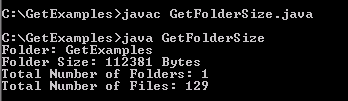Tutorials

# Java get Folder Size

In this example you will learn In this section, you will learn how to get the size of specified folder.

In this example you will learn In this section, you will learn how to get the size of specified folder.

# Java get Folder Size

In this section, you will learn how to get the size of specified folder.

In the given example, we are going to show you the size of specified folder and also the number of files and folders that are presented in the specified folder. We have create a method getFileSize() in order to obtain the size of folder. The totalFolder++ counts the total folders and totalFile++ counts the total files.

Here is the video tutorial of "How to get Folder Size in Java?":

folder.getName()-This method returns the folder name.

folder.listFiles()- This method returns an array of files presented in the specified folder.

filelist[i].length() - This method returns the length of files presented in the specified folder.

Following code will calculate the size of folder in bytes.

 long fileSizeByte=size.getFileSize(new File(folder))

Here is the code of GetFolderSize.java

 ``` import java.io.File; import java.text.DecimalFormat; import java.text.NumberFormat; public class GetFolderSize {   int totalFolder=0;   int totalFile=0;   public static void main(String args [])   {     String folder = "C:/GetExamples";   try{   GetFolderSize size=new GetFolderSize();   long fileSizeByte=size.getFileSize(new File(folder));   System.out.println("Folder Size: "+fileSizeByte+" Bytes" );   System.out.println("Total Number of Folders: "+size.getTotalFolder());   System.out.println("Total Number of Files: "+size.getTotalFile());   }catch (Exception e)   {}   }   public long getFileSize(File folder) {   totalFolder++;    System.out.println("Folder: " + folder.getName());   long foldersize = 0;   File[] filelist = folder.listFiles();   for (int i = 0; i < filelist.length; i++) {   if (filelist[i].isDirectory()) {   foldersize += getFileSize(filelist[i]);   } else {   totalFile++;   foldersize += filelist[i].length();   }   }   return foldersize;   }   public int getTotalFolder() {   return totalFolder;   }   public int getTotalFile() {   return totalFile;   } }```

Output will be displayed as: